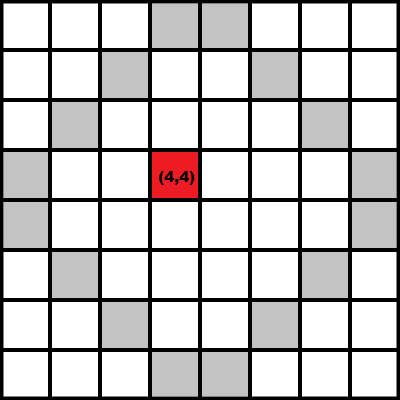# Flood Fill Algorithm

Flood fill is an algorithm mainly used to determine a bounded area connected to a given node in a multi-dimensional array. It is a close resemblance to the bucket tool in paint programs.

The most approached implementation of the algorithm is a stack-based recursive function, and that’s what we’re gonna talk about next.

### How does it work?

The problem is pretty simple and usually follows these steps:

1. Take the position of the starting point.
2. Decide whether you want to go in 4 directions ( N, S, W, E ) or 8 directions ( N, S, W, E, NW, NE, SW, SE ).
3. Choose a replacement color and a target color.
4. Travel in those directions.
5. If the tile you land on is a target, replace it with the chosen color.
6. Repeat 4 and 5 until you’ve been everywhere within the boundaries.

Let’s take the following array as an example:The red square is the starting point and the gray squares are the so called walls.

For further details, here’s a piece of code describing the function:

``````int wall = -1;

void flood_fill(int pos_x, int pos_y, int target_color, int color)
{

if(a[pos_x][pos_y] == wall || a[pos_x][pos_y] == color) // if there is no wall or if i haven't been there

if(a[pos_x][pos_y] != target_color) // if it's not color go back
return;

a[pos_x][pos_y] = color; // mark the point so that I know if I passed through it.

flood_fill(pos_x + 1, pos_y, color);  // then i can either go south
flood_fill(pos_x - 1, pos_y, color);  // or north
flood_fill(pos_x, pos_y + 1, color);  // or east
flood_fill(pos_x, pos_y - 1, color);  // or west

return;

}
``````

As seen above, my starting point is (4,4). After calling the function for the start coordinates x = 4 and y = 4 , I can start checking if there is no wall or color on the spot. If that is valid i mark the spot with one “color” and start checking the other adjacent squares.

Going south we will get to point (5,4) and the function runs again.

### Exercise problem

I always considered that solving a (or more) problem/s using a newly learned algorithm is the best way to fully understand the concept.

So here’s one:

Statement:

In a bidimensional array you are given n number of “islands” . Try to find the largest area of an island and the corresponding island number. 0 marks water and any other x between 1 and n marks one square from the surface corresponding to island x.

Input

• n - the number of islands.
• l,c - the dimensions of the matrix.
• the next l lines, c numbers giving the l th row of the matrix.

Output

• i - the number of the island with the largest area.
• A - the area of the i 'th island.

Ex:

You have the following input:

``````2 4 4
0 0 0 1
0 0 1 1
0 0 0 2
2 2 2 2
``````

For which you will get island no. 2 as the biggest island with the area of 5 squares.

### Hints

The problem is quite easy, but here are some hints:

``````1. Use the flood-fill algorithm whenever you encounter a new island.
2. As opposed to the sample code, you should go through the area of the island and not on the ocean (0 tiles).
``````